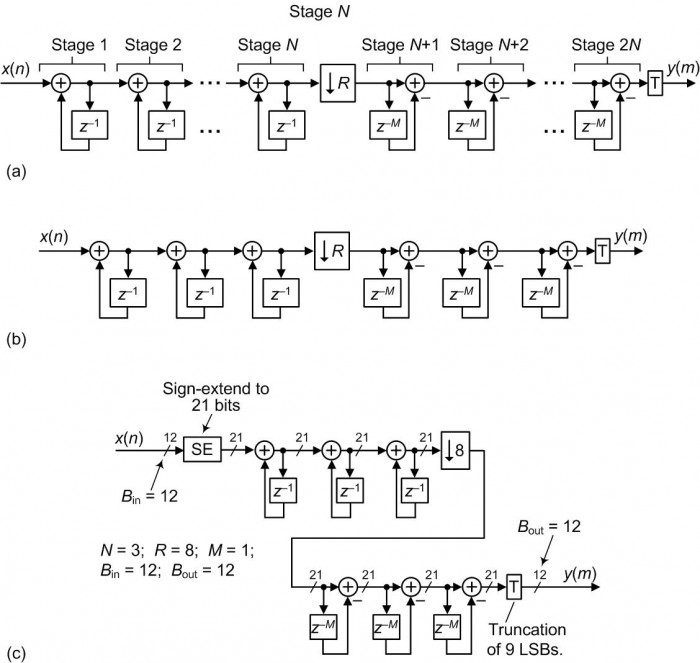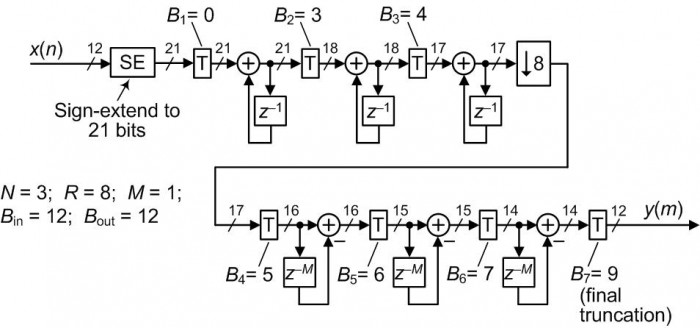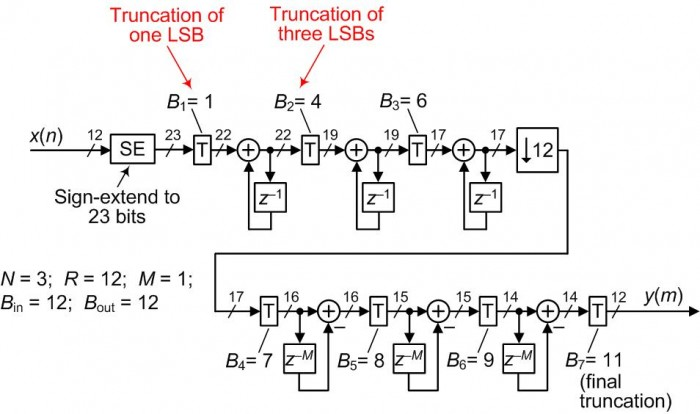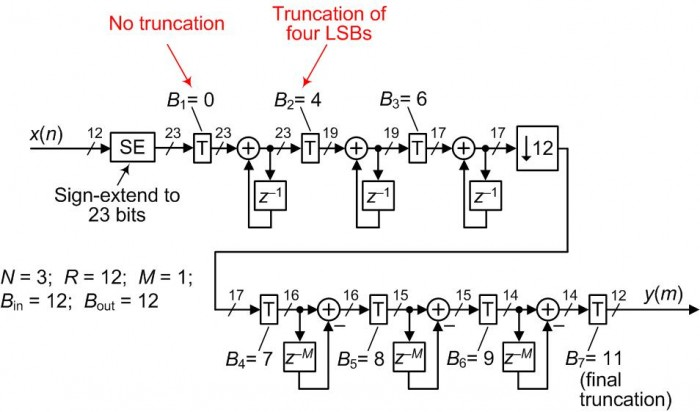## Computing CIC Filter Register Pruning Using Matlab [Updated]

February 15, 20128 comments Coded in Matlab

The following Matlab code computes the amount register bit-pruning described in Eugene's Hogenauer's famous paper on cascaded integrator-comb (CIC) decimation filters . Although there are many detailed descriptions of CIC filters in the literature [2-6], my notation here corresponds to that used in Hogenauer's IEEE paper.

Figure 1(a) shows a general Nth-order CIC filter and Figure 1(b) shows an example of a 3rd-order CIC filter.Figure 1(c) shows that filter having the following parameters: N=3; R=8; M=1; Bin=12; andBout=12. (The variables Bin and Bout represent the filter's input and output binary word widths respectively.) Due to those parameters, the filter's gain is 512 and all of the stages' adder/accumulator registers must be 21 bits in width.With the output word width being 12 bits, the 'T' block in Figure 1(c) represents a truncation of nine least significant bits (LSBs).

Note in Figure 1(c) that the 12-bit input word, in two's complement format, must be sign-extended (SE) to 21 bits before it's applied to the adder in the 1st integrator.Figure 1.

While Hogenauer's IEEE CIC paper was certainly revolutionary, I believe one of the truly clever aspects of his paper was how he addressed that final 'T' truncation operation. Hogenauer realized that the quantization error induced by that final truncation can be distributed among the individual stages of the filter by truncating LSBs from each stage's adder/accumulator output word. In doing so, he could reduce the stages' computational workloads without increasing the overallfilter truncation quantization error. Hogenauer's description of this whole idea is a bit challenging to understand but it's certainly not beyond comprehension. (I had to read his 'distributed truncation' text several times.)

Figure 2 shows an example of the 'distributed truncation', what's called "register pruning", for the filter in Figure 1(c). The variable Bj specifies the number of adder/accumulator LSBs can be truncated from the Figure 1(c) filter's jth stage. For example, three LSBs are truncated (loped off) from the output of the first integrator's 21-bit adder/accumulator output word prior to routing to the second integrator. Therefore the second integrator in Figure 2 need only accept an 18-bit input word rather than a 21-bit input word as was the case in Figure 1(c).Figure 2.

The following Matlab code computes the values for Bj for the jth stages in Nth-order CIC decimation filters where j = 1, 2, ...2N+1. Note that B2N+1 represents the final truncation taking place after the final comb stage.

Running the following Matlab code for the Figure 1(c) filter parameters yields a result of:The Bj values in the above center column are the Bj values shown in Figure 2.

Warning: It's possible, for certain combinations of N, R, M, Bin, and Bout, that negative values of Bj are computed, particularly when the product RM is a small number, and Bout>Bin. When using the following Matlab code, if you compute a negative value of Bj then that value should be ignored and no truncation should performed at that jth stage.

UPDATE Jan. 23, 2022

Based on the Jan., 2022 Comments to my CIC filter blog at:

I have learned something both interesting and important regarding register pruning of decimating CIC filters. Depending on the filter's values for N and R, it is quite likely for Hogenaurer's pruning method to recommend an initial truncation of one bit of the filter's input sequence prior to the first integrator. For example, this occurs when N = 3 and R = 12 causing B1 = 1 as shown below in Figure 3.Figure 3.

The Figure 3 situation troubles me. Reducing an input sequence's bit width by one bit would, unfortunately, decrease that sequence's signal-to-quantization noise ratio by 6 dB prior to any filtering.

So, if pruning the accumulator registers in a decimating CIC filter calls for a nonzero value for B1, I suggest you set B1 = 0 (no truncation) and increase the truncation following the first integrator by one additional LSB as shown in Figure 4.Figure 4.

References

 Eugene Hogenauer, "An Economical Class of Digital Filters For Decimation and Interpolation," IEEE Trans. Acoust. Speech and Signal Proc., Vol. ASSP?29, April 1981, pp. 155-162.

 Richard Lyons, "A Beginner's Guide To Cascaded Integrator-Comb (CIC) Filters", March, 2020. Available at:

 F. Harris, Multirate Signal Processing for Communication Systems, Prentice Hall, Upper Saddle River, New Jersey, 2004, Chapter 11.

 Richard Lyons, Understanding Digital Signal Processing, 3rd Ed., Prentice Hall, Upper Saddle River, New Jersey, 2010, Chapter 10.

 Peter Thorwartl, "Implementation of Filters", Part 3, lecture notes. Available at:

should be:

 Uwe Meyer-Baese, "Digital Signal Processing with Field Programmable Gate Arrays"3rd Ed., Springer Publishing, 2007, Section 5.3.4.

Matlab Code:

Here's the Matlab code that computes CIC decimation filter register pruning

for up to 7th-order filters:

``````%  Filename: CIC_Word_Truncation.m
%
%  Computes CIC decimation filter accumulator register
%  truncation in each filter stage based on Hogenauer's
%  'accumulator register pruning' technique.
%
%   Inputs:
%     N = number of decimation CIC filter stages (filter order).
%     R = CIC filter rate change factor (decimation factor).
%     M = differential delay.
%     Bin = number of bits in an input data word.
%     Bout = number of bits in the filter's final output data word.
%   Outputs:
%     Stage number (ranges from 1 -to- 2*N+1).
%     Bj = number of least significant bits that can be truncated
%       at the input of a filter stage.
%     Accumulator widths = number of a stage's necessary accumulator
%       bits accounting for truncation.

%  Richard Lyons Feb., 2012

clear, clc

%%%%%%%%%%%%%%%%%%%%%%%%%%%%%%%%%%%%%%%%%%%%%%%%%
%  Define CIC filter parameters
%%%%%%%%%%%%%%%%%%%%%%%%%%%%%%%%%%%%%%%%%%%%%%%%%
%N = 4;  R = 25;  M = 1;  Bin = 16;  Bout = 16; % Hogenauer paper, pp. 159
%N = 3;  R = 32;  M = 2;  Bin = 8;  Bout = 10; % Meyer Baese book, pp. 268
%N = 3;  R = 16;  M = 1;  Bin = 16;  Bout = 16; % Thorwartl's PDF file

N = 3; R = 8; M = 1; Bin = 12; Bout = 12; % Lyons' blog Figure 2 example

%%%%%%%%%%%%%%%%%%%%%%%%%%%%%%%%%%%%%%%%%%
% Find h_sub_j and "F_sub_j" values for (N-1) cascaded integrators
%%%%%%%%%%%%%%%%%%%%%%%%%%%%%%%%%%%%%%%%%%
disp(' ')
for j = N-1:-1:1
h_sub_j = [];
h_sub_j((R*M-1)*N + j -1 + 1) = 0;
for k = 0:(R*M-1)*N + j -1
for L = 0:floor(k/(R*M)) % Use uppercase "L" for loop variable
Change_to_Result = (-1)^L*nchoosek(N, L)...
*nchoosek(N-j+k-R*M*L,k-R*M*L);
h_sub_j(k+1) =  h_sub_j(k+1) + Change_to_Result;
end % End "L" loop
end % End "k" loop
F_sub_j(j) = sqrt(sum(h_sub_j.^2));
end % End "j" loop
%%%%%%%%%%%%%%%%%%%%%%%%%%%%%%%%%%%%%%%%%%%%%%%%%

%%%%%%%%%%%%%%%%%%%%%%%%%%%%%%%%%%%%%%%%%%%%%%%%%
% Define "F_sub_j" values for up to seven cascaded combs
%%%%%%%%%%%%%%%%%%%%%%%%%%%%%%%%%%%%%%%%%%%%%%%%%
F_sub_j_for_many_combs = sqrt([2, 6, 20, 70, 252, 924, 3432]);
%%%%%%%%%%%%%%%%%%%%%%%%%%%%%%%%%%%%%%%%%%%%%%%%%

%%%%%%%%%%%%%%%%%%%%%%%%%%%%%%%%%%%%%%%%%%%%%%%%%
%  Compute F_sub_j for last integrator stage
%%%%%%%%%%%%%%%%%%%%%%%%%%%%%%%%%%%%%%%%%%%%%%%%%
F_sub_j(N) = F_sub_j_for_many_combs(N-1)*sqrt(R*M);  % Last integrator
%%%%%%%%%%%%%%%%%%%%%%%%%%%%%%%%%%%%%%%%%%%%%%%%%

%%%%%%%%%%%%%%%%%%%%%%%%%%%%%%%%%%%%%%%%%%%%%%%%%
%  Compute F_sub_j for N cascaded filter's comb stages
%%%%%%%%%%%%%%%%%%%%%%%%%%%%%%%%%%%%%%%%%%%%%%%%%
for j = 2*N:-1:N+1
F_sub_j(j) = F_sub_j_for_many_combs(2*N -j + 1);
end
%%%%%%%%%%%%%%%%%%%%%%%%%%%%%%%%%%%%%%%%%%%%%%%%%

%%%%%%%%%%%%%%%%%%%%%%%%%%%%%%%%%%%%%%%%%%%%%%%%%
% Define "F_sub_j" values for the final output register truncation
%%%%%%%%%%%%%%%%%%%%%%%%%%%%%%%%%%%%%%%%%%%%%%%%%
F_sub_j(2*N+1) = 1; % Final output register truncation
%%%%%%%%%%%%%%%%%%%%%%%%%%%%%%%%%%%%%%%%%%%%%%%%%

%%%%%%%%%%%%%%%%%%%%%%%%%%%%%%%%%%%%%%%%%%%%%%%%%
% Compute column vector of minus log base 2 of "F_sub_j" values
%%%%%%%%%%%%%%%%%%%%%%%%%%%%%%%%%%%%%%%%%%%%%%%%%
Minus_log2_of_F_sub_j = -log2(F_sub_j)';
%%%%%%%%%%%%%%%%%%%%%%%%%%%%%%%%%%%%%%%%%%%%%%%%%

%%%%%%%%%%%%%%%%%%%%%%%%%%%%%%%%%%%%%%%%%%%%%%%%%
% Compute total "Output_Truncation_Noise_Variance" terms
%%%%%%%%%%%%%%%%%%%%%%%%%%%%%%%%%%%%%%%%%%%%%%%%%
CIC_Filter_Gain = (R*M)^N;
Num_of_Bits_Growth = ceil(log2(CIC_Filter_Gain));
% The following is from Hogenauer's Eq. (11)
%Num_Output_Bits_With_No_Truncation = Num_of_Bits_Growth +Bin -1;
Num_Output_Bits_With_No_Truncation = Num_of_Bits_Growth +Bin;
Num_of_Output_Bits_Truncated = Num_Output_Bits_With_No_Truncation -Bout;
Output_Truncation_Noise_Variance = (2^Num_of_Output_Bits_Truncated)^2/12;
%%%%%%%%%%%%%%%%%%%%%%%%%%%%%%%%%%%%%%%%%%%%%%%%%

%%%%%%%%%%%%%%%%%%%%%%%%%%%%%%%%%%%%%%%%%%%%%%%%%
% Compute log base 2 of "Output_Truncation_Noise_Standard_Deviation" terms
%%%%%%%%%%%%%%%%%%%%%%%%%%%%%%%%%%%%%%%%%%%%%%%%%
Output_Truncation_Noise_Standard_Deviation = ...
sqrt(Output_Truncation_Noise_Variance);
Log_base2_of_Output_Truncation_Noise_Standard_Deviation = ...
log2(Output_Truncation_Noise_Standard_Deviation);
%%%%%%%%%%%%%%%%%%%%%%%%%%%%%%%%%%%%%%%%%%%%%%%%%

%%%%%%%%%%%%%%%%%%%%%%%%%%%%%%%%%%%%%%%%%%%%%%%%%
% Compute column vector of "half log base 2 of 6/N" terms
%%%%%%%%%%%%%%%%%%%%%%%%%%%%%%%%%%%%%%%%%%%%%%%%%
Half_Log_Base2_of_6_over_N = 0.5*log2(6/N);
%%%%%%%%%%%%%%%%%%%%%%%%%%%%%%%%%%%%%%%%%%%%%%%%%

%%%%%%%%%%%%%%%%%%%%%%%%%%%%%%%%%%%%%%%%%%%%%%%%%
% Compute desired "B_sub_j" vector
%%%%%%%%%%%%%%%%%%%%%%%%%%%%%%%%%%%%%%%%%%%%%%%%%
B_sub_j = floor(Minus_log2_of_F_sub_j ...
+ Log_base2_of_Output_Truncation_Noise_Standard_Deviation ...
+ Half_Log_Base2_of_6_over_N);
%%%%%%%%%%%%%%%%%%%%%%%%%%%%%%%%%%%%%%%%%%%%%%%%%
disp(' '), disp(' ')
disp(['N = ',num2str(N),',   R = ',num2str(R),',   M = ',num2str(M),...
',   Bin = ', num2str(Bin),',   Bout = ',num2str(Bout)])
disp(['Num of Bits Growth Due To CIC Filter Gain = ', ...
num2str(Num_of_Bits_Growth)])
disp(['Num of Accumulator Bits With No Truncation = ', ...
num2str(Num_Output_Bits_With_No_Truncation)])
% disp(['Output Truncation Noise Variance = ', ...
%     num2str(Output_Truncation_Noise_Variance)])
% disp(['Log Base2 of Output Truncation Noise Standard Deviation = ',...
%         num2str(Log_base2_of_Output_Truncation_Noise_Standard_Deviation)])
% disp(['Half Log Base2 of 6/N = ', num2str(Half_Log_Base2_of_6_over_N)])
%%%%%%%%%%%%%%%%%%%%%%%%%%%%%%%%%%%%%%%%%%%%%%%%%
% Create and display "Results" matrix
%%%%%%%%%%%%%%%%%%%%%%%%%%%%%%%%%%%%%%%%%%%%%%%%%
for Stage = 1:2*N
Results(Stage,1) = Stage;
Results(Stage,2) = F_sub_j(Stage);
Results(Stage,3) = Minus_log2_of_F_sub_j(Stage);
Results(Stage,4) = B_sub_j(Stage);
Results(Stage,5) = Num_Output_Bits_With_No_Truncation -B_sub_j(Stage);
end
% Include final output stage truncation in "Results" matrix
Results(2*N+1,1) = 2*N+1;  % Output stage number
Results(2*N+1,2) = 1;
Results(2*N+1,4) = Num_of_Output_Bits_Truncated;
Results(2*N+1,5) = Bout;
%Results % Display "Results" matrix in raw float-pt.form

% % Display "F_sub_j" values if you wish
% disp(' ')
% disp(' Stage        Fj        -log2(Fj)    Bj   Accum width')
% for Stage = 1:2*N+1
%   disp(['  ',sprintf('%2.2g',Results(Stage,1)),sprintf('\t'),sprintf('%12.3g',Results(Stage,2)),...
%         sprintf('\t'),sprintf('%7.5g',Results(Stage,3)),sprintf('\t'),...
%         sprintf('%7.5g',Results(Stage,4)),sprintf('\t'),sprintf('%7.5g',Results(Stage,5))])
% end

% Display Stage number, # of truncated input bits, & Accumulator word widths
disp(' ')
disp(' Stage(j)   Bj   Accum (adder) width')
for Stage = 1:2*N
disp(['  ',sprintf('%2.0f',Results(Stage,1)),...
sprintf('\t'),...
sprintf('%5.5g',Results(Stage,4)),sprintf('\t'),...
sprintf('%7.5g',Results(Stage,5))])
end
disp(['  ',sprintf('%2.0f',Results(2*N+1,1)),...
sprintf('\t'),...
sprintf('%5.5g',Results(2*N+1,4)),sprintf('\t'),...
sprintf('%7.5g',Results(2*N+1,5)),' (final truncation)'])
%%%%%%%%%%%%%%%%%%%%%%%%%%%%%%%%%%%%%%%%%%%%%%%%%``````